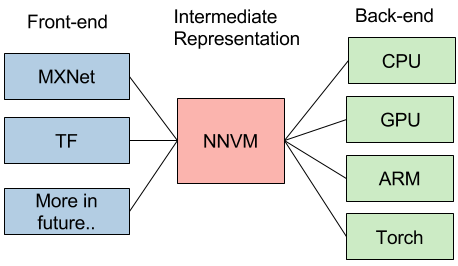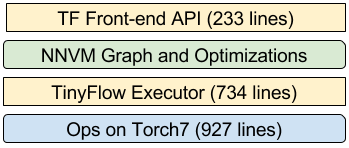Want to share your content on R-bloggers? click here if you have a blog, or here if you don't.

TL;DR Do something fun, How about build your own TensorFlow with NNVM and Torch7

This is a new interesting era of deep learning, with emergence trend of new system, hardware and computational model. The usecase for deep learning is more heterogeneous, and we need tailored learning system for our cars, mobiles and cloud services. The future of deep learning system is going to be more heterogeneous, and we will find emergence need of different front-ends, backends and optimization techniques. Instead of building a monolithic solution to solve all these problems, how about adopt unix philosophy, build effective modules for learning system, and assemble them together to build minimum and effective systems?

We opensourced NNVM library a few weeks ago, as a step to build re-usable modules along multiple deep learning systems. Specifically, NNVM provides a common way to represent and optimize a computation graph and target multiple front-backends.This sounds great, you may say, how about give a concrete example? Of course any reasonable project need example code. Since NNVM is a library to help building deep learning systems, why not provide an example on building something real? So here comes TinyFlow. Let us try something fun in this post and introduce how one can build a new deep learning system with NNVM. To put it short, TinyFlow is an “example code” for NNVM. It is of course a fully functioning deep learning system and even has some features that is not currently available in TensorFlow.

The entire project takes around 2K lines of code, and can run the example code like the followings. You will find that everything is same as TensorFlow, except that in the first line, we import tinyflow instead.

```import tinyflow as tf
from tinyflow.datasets import get_mnist

# Create the model
x = tf.placeholder(tf.float32, [None, 784])
W = tf.Variable(tf.zeros([784, 10]))
y = tf.nn.softmax(tf.matmul(x, W))

# Define loss and optimizer
y_ = tf.placeholder(tf.float32, [None, 10])

cross_entropy = tf.reduce_mean(-tf.reduce_sum(y_ * tf.log(y), reduction_indices=))

sess = tf.Session()
sess.run(tf.initialize_all_variables())

# get the mnist dataset
mnist = get_mnist(flatten=True, onehot=True)

for i in range(1000):
batch_xs, batch_ys = mnist.train.next_batch(100)
sess.run(train_step, feed_dict={x: batch_xs, y_:batch_ys})

correct_prediction = tf.equal(tf.argmax(y,1), tf.argmax(y_,1))
accuracy = tf.reduce_mean(correct_prediction)

print(sess.run(accuracy, feed_dict={x: mnist.test.images, y_: mnist.test.labels}))

```

## How do we do it

In order to build such a system, there are several major things we need to do:

• Build a front-end that can compose and execute computation graph like TensorFlow do.
• Support APIs such as shape inference, automatic differentiation.
• Write a graph execution engine. This is where many system optimizations need to come, current optimizations mainly include static shape inference and memory saving optimization.
• Implement specific operators such as convolution, matrix multiplication.

Two major ingredients are used to simplify the development of TinyFlow – NNVM is used to build front-end, and provide common library to do graph optimization, differentiation and memory optimizations – Torch7 is used as a dependency to quickly implement the operators we need.

The following is a code snippet we use to register the multiplication operators in TinyFlow. FLuaCompute registers a piece of lua function that uses torch to carry out the real computation of the operator.

```NNVM_REGISTER_OP(mul)
.describe("elementwise multiplication of two variables")
.set_num_inputs(2)
.set_attr<FInferShape>("FInferShape", SameShape)
.set_attr<FInplaceOption>("FInplaceOption", InplaceIn0Out0)
.set_attr<FLuaCompute>(
"FLuaCompute", R"(
function(x, y, kwarg)
return function()
torch.cmul(y, x, x)
end
end
)")
return std::vector<NodeEntry>{
};
});

```

### Why Ops from Torch

For a mature deep learning system like Tensorflow, MXNet, Caffe. The operators are usually implemented in C++. This consumes a major amount of effort. Since TinyFlow is a demonstration project on how deep learning system can be built, we choose Torch7 because it provides a rather complete set of operations and can be embedded into C++ backend with low cost. We also intentionally choose to avoid using MXNet as backend, since MXNet already uses NNVM as intermediate layer, and it would be more fun to try something different. The general design itself, however, does not bind the system to be Lua specific, and can execute other types of operators.

It is actually also not a bad idea to embed Lua code for customized operators, so that we can reuse common piece that are available in the Torch community. We have provided a Torch plugin in MXNet and it is quite helpful to some of our users.

### The System Stack

Having operators is only part of the story, we still need to stitch the computation together, and provide code for shape/type inference and memory sharing. The functions such as FInferShape and FInplaceOption is registered to provide these information, then we reuse the memory optimization and shape/type inference module in NNVM to write the execution backend. This is what the system stack looks like in TinyFlow.## Interesting Features that are not in TensorFlow

### Automatic Variable Shape Inference

One of the main pain point I had when using TF API is that we have to initialize the variables by giving their shape. This is quite inconvenient especially for deep networks, where the shape of weights in later layers depend on previous inputs. By using the shape inference module in TinyFlow, we provide a simple new API like the automatic variable shape inference provided in MXNet

```x = tf.placeholder(tf.float32)
fc1 = tf.nn.linear(x, num_hidden=100, name="fc1", no_bias=False)
relu1 = tf.nn.relu(fc1)
fc2 = tf.nn.linear(relu1, num_hidden=10, name="fc2")

# define loss
label = tf.placeholder(tf.float32)
cross_entropy = tf.nn.mean_sparse_softmax_cross_entropy_with_logits(fc2, label)

# Automatic variable shape inference API, infers the shape and initialize the weights.
known_shape = {x: [100, 28 * 28], label: }
init_step = []
for v, name, shape in tf.infer_variable_shapes(
cross_entropy, feed_dict=known_shape):
init_step.append(tf.assign(v, tf.normal(shape)))
print("shape[%s]=%s" % (name, str(shape)))
sess.run(init_step)
```

The idea is to provide the shape hints such as number of hidden layers in the operators, enable automatic creation of weight variables in these operators, and use `tf.infer_variable_shapes` to discover the shape of the variables in a network.

All these features are provided by NNVM modules and we only need to expose them via the API.

### Static Memory Planning

TensorFlow uses a dynamic memory allocator to manage memory because it need to handle too general cases of complicated graphs including loop, condition. Many use-cases in deep learning does not need these, and a fixed computation graph can be optimized in a more static way. TinyFlow uses NNVM’s memory optimization module, which provides inplace manipulation and automatic memory sharing. This leads to similar memory saving behavior as in MXNet which allows training of larger models with limited resources. We could also add more interesting features such as sublinear gradient graph planning as described in https://arxiv.org/abs/1604.06174 to futher reduce the memory cost.

## Modular Library for Deep Learning System Building

We do not build every piece of TinyFlow from scratch. Instead, we explore how we can reuse common modules in deep learning systems such as operators from Torch7 and graph representation/optimizations from NNVM to quickly build a real deep learning system. We believe that such reusability and modularization in deep learning can help us to advance system faster, and build minimum but powerful system by putting useful parts together.

So that the improvements we made to TinyFlow and NNVM not only affect this project, but also all the projects that uses NNVM as intermediate representation layer. We believe more module reuse between deep learning systems like NNVM would happen to move learning system building faster as a whole ecosystem.

### Tutorial on How to Build Deep Learning System

We intentionally keep the codebase small. However, it still elaborate many key concepts and optimizations that exists in major deep learning systems, such as computation graph, automatic differentiation, shape/type inference, memory optimization. TinyFlow is a perfect material to explain these concepts and teach students about how to build new deep learning systems.